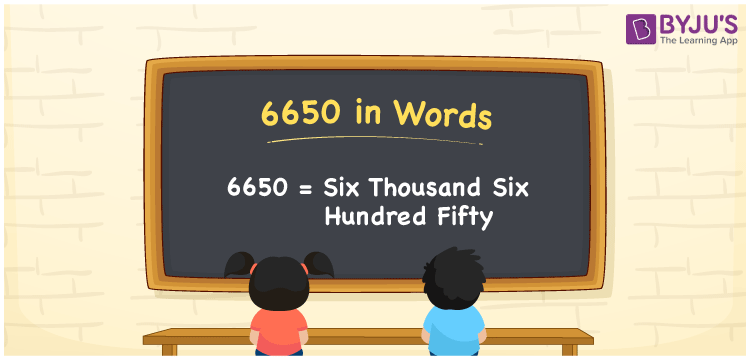# 6650 in words

6650 in words is written as Six Thousand Six Hundred and Fifty. 6650 represents the count or value. The article on Counting Numbers can give you an idea about count or counting. The number 6650 is used in expressions that relate to money, distance, length, area and others.

Let us consider an example for 6650. “That house is so big. It is Six Thousand Six Hundred and Fifty square meters in area.”

 6650 in words Six Thousand Six Hundred and Fifty Six Thousand Six Hundred and Fifty in Numbers 6650

## 6650 in English Words## How to Write 6650 in Words?

We can convert 6650 to words using a place value chart. The number 6650 has 4 digits, so let’s make a chart that shows the place value up to 4 digits.

 Thousands Hundreds Tens Ones 6 6 5 0

Thus, we can write the expanded form as:

6 × Thousand + 6 × Hundred + 5 × Ten + 0 × One

= 6 × 1000 + 6 × 100 + 5 × 10 + 0 × 1

= 6650

= Six Thousand Six Hundred and Fifty.

6650 is the natural number that is succeeded by 6649 and preceded by 6651.

6650 in words – Six Thousand Six Hundred and Fifty.

Is 6650 an odd number? – No.

Is 6650 an even number? – Yes.

Is 6650 a perfect square number? – No.

Is 6650 a perfect cube number? – No.

Is 6650 a prime number? – No.

Is 6650 a composite number? – Yes.

## Solved Example

1. Write the number 6650 in expanded form

Solution: 6 × 1000 + 6 × 100 + 5 × 10 + 0 × 1

We can write 6650 = 6000 + 600 + 50 + 0

= 6 × 1000 + 6 × 100 + 5 × 10 + 0 × 1

## Frequently Asked Questions on 6650 in words

Q1

### How to write 6650 in words?

6650 in words is written as Six Thousand Six Hundred and Fifty.
Q2

### Is 6650 divisible by 2?

Yes. 6650 is divisible by 2.
Q3

### Is 6650 a perfect cube number?

No. 6650 is not a perfect cube number.